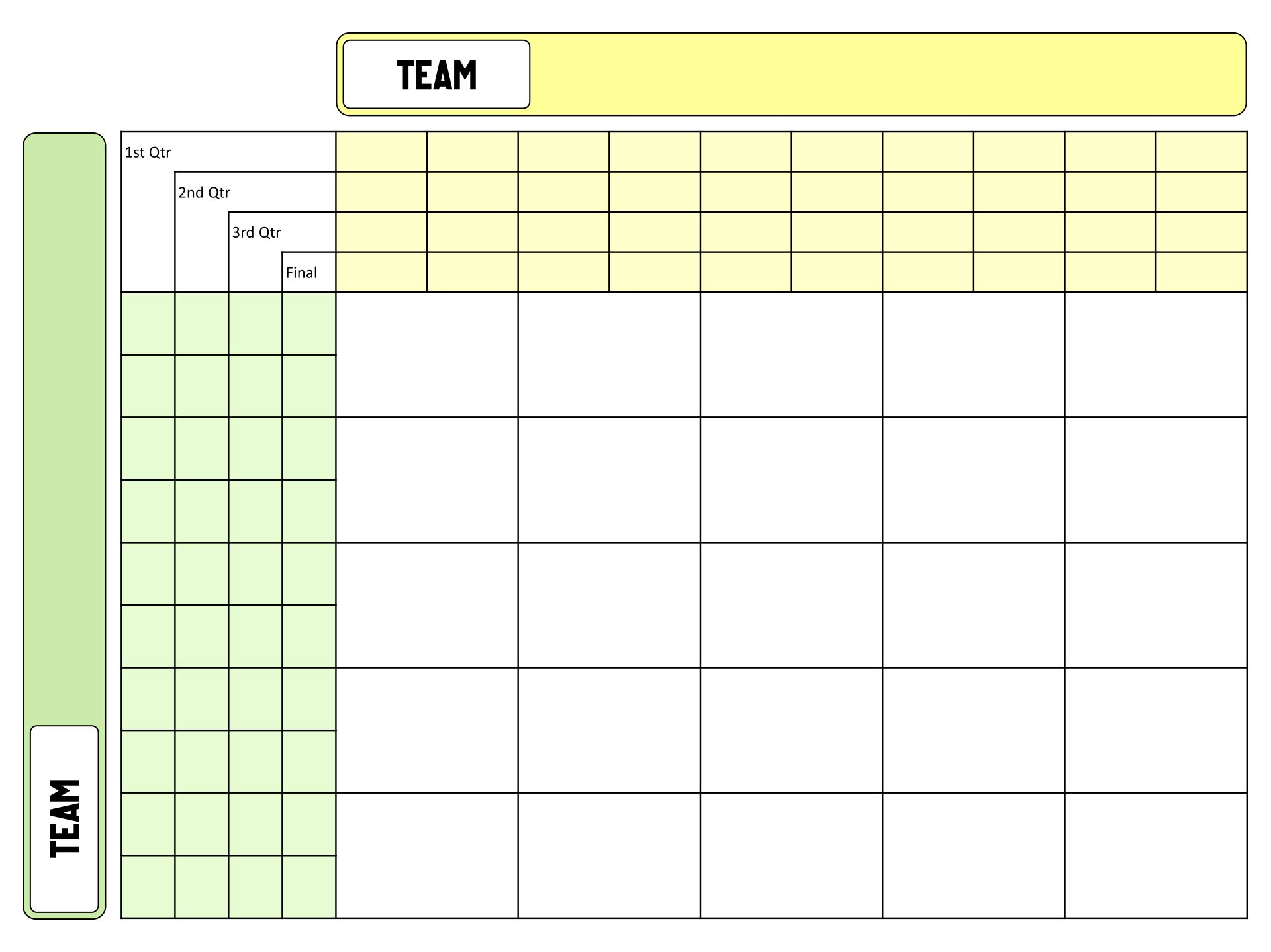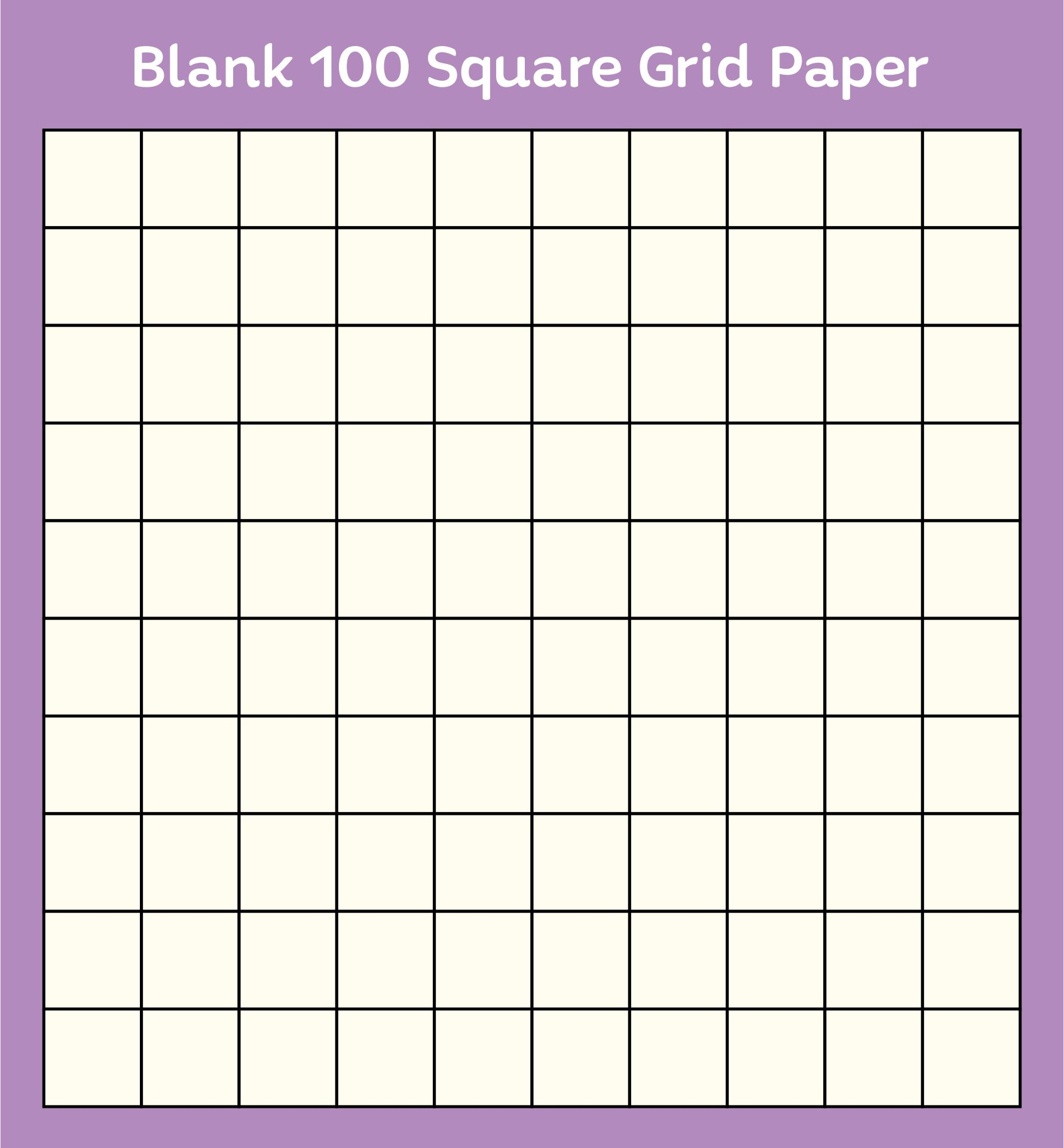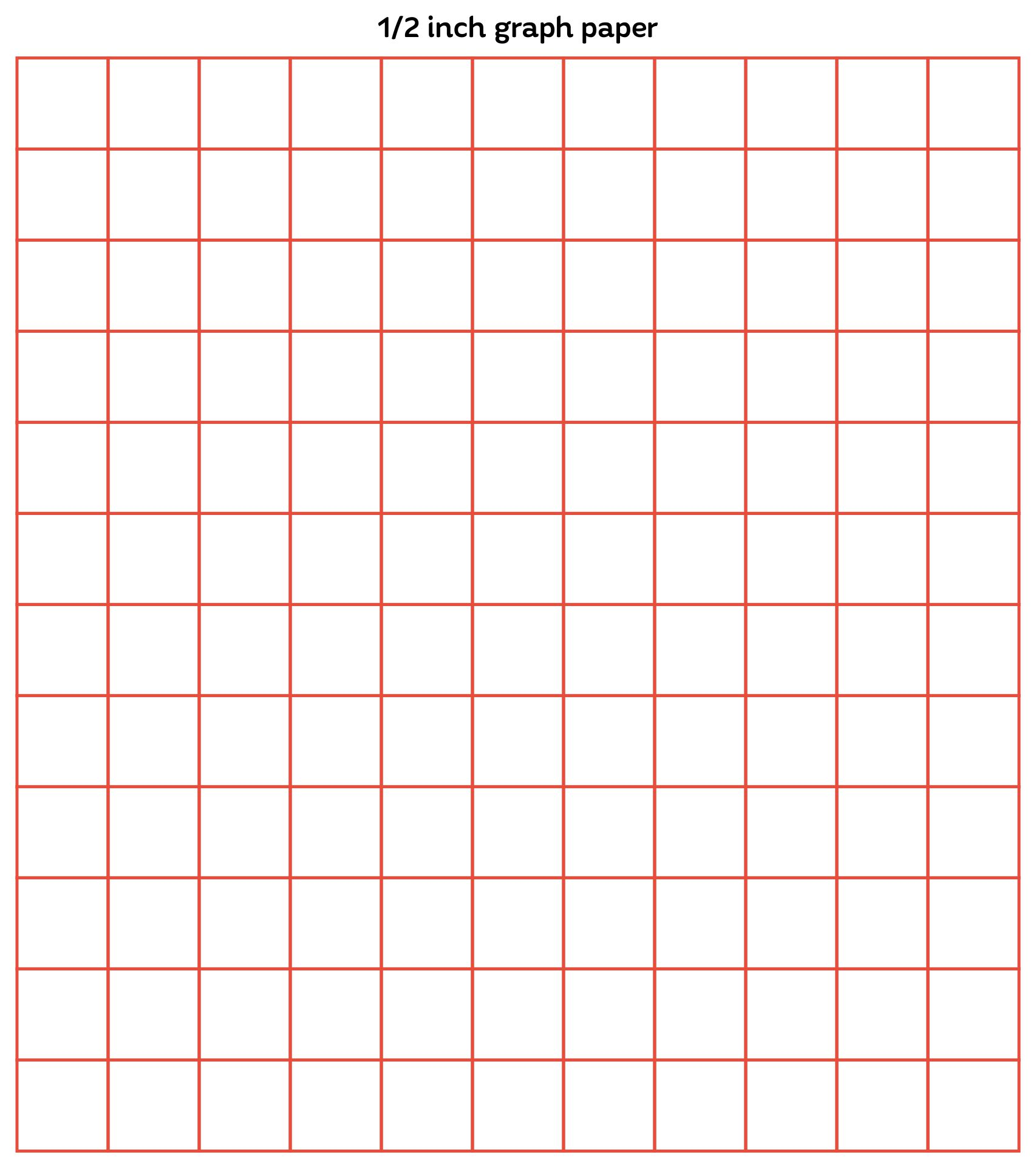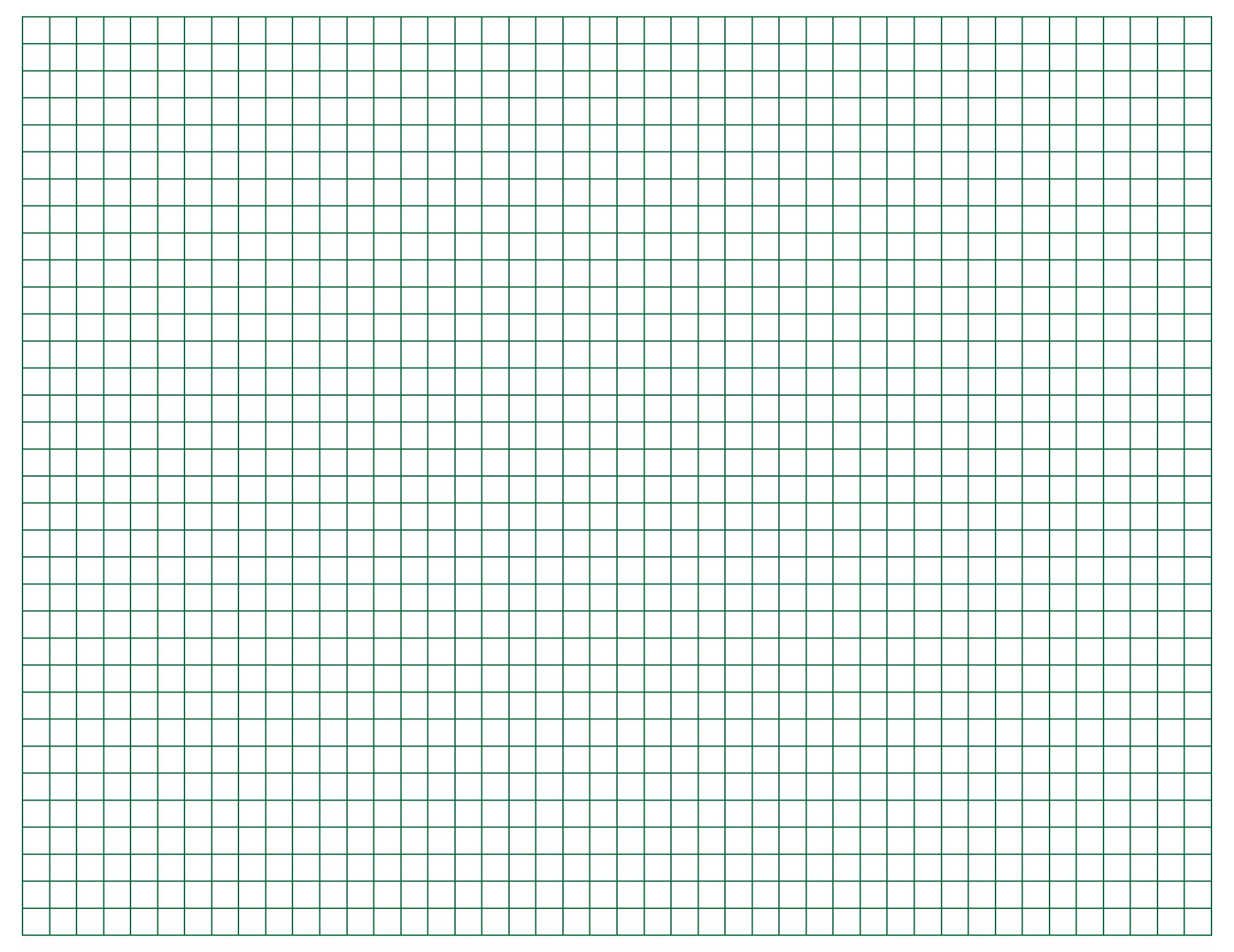# Printable Grid 4 X 5OtherMay 18, 2022By PrintableeNo Comment### How do you use the grid paper in math?

Counting using grid paper itself is being taught during elementary school. Besides understanding how the positive and negative numbers work, there is more to observe in using graph paper as a math understanding tool. Since each student has difficulty in absorbing the lesson given as many other subjects, as for math problem solving, grid paper can be an accommodation for students to solve not only the basic math operations but also multi-digit multiplication.

It also can be used to teach the coordinate planes or the grid plane where it is being used to count with the coordinate on its axes (X-axis and Y-axis). In the real-life application, this can be helpful to understand how to read graphs or charts which refers to the basic vision of math to understand patterns and help develop problem-solving skills.  In math terms, the small unit squares on the graph paper are called cells and the line that is subdivided into small unit segments is what can be called the bars. The grid lines can be a tool to help students with counting with the basic notion of how they are able to describe and find the answers by counting with grid point (node)s in various rectangle dimensions. As an example, a rectangle Y with 2 x 5 dimensions has 18 grid points. The answer lies in the points within the rectangle being counted. Among many other counting exercises in math with grid paper, you can try this number points counting as a beginner starter.

### What are some types of early visual models in mathematics?

Before the new modern math started, back in the days the practice of math came with pictures and symbols that looked much more familiar and easy to follow to communicate such as fingers. Until today, the application is timeless as today most of us will definitely count using our fingers and recite the numbers, especially children. It also developed within each region within the whole country. The Romans used to make connections between fingers, such as the V hand showing the number 5 and when each of the thumbs was arranged, as if you wanted to make a bird, it represented X (ten) for the Romans for their everyday needs. On the other side, the Egyptian numerals were likely represented with symbols such as the number ten is shown as a cattle hobble. It is also very much a big use because they can easily mark with lines and more everyday objects for greater numbers. For today, the practical use of counting through visual models was applied to more variety such as using beads that were known to be introduced by Maria Montessori in the 1900s in Italy.We also have more printable other you may like:
Printable 5x5 Grid
Printable Observation Forms
Despicable Me Minion Mouth Printable### What is the difference between graphs and charts?

Graphs and charts were visibly similar to be noticed. However, both of them were still different in various aspects. From the meaning, a chart is used to show information in the form of a diagram, table, or graph. However, it is used to compress large amounts of information in one format. Meanwhile, a graph is a kind of chart created with a basic set of math relations in the sets with axes (X-axis and Y-axis). Charts are more common to use when you are about to represent data for business and survey results. The graph is used to show exact numbers and raw data as to bring more rational figures within its points and lines. Manually, you can use grid paper to write and draw the data easier within the lines and cells. The reason why graphs and charts were pretty much being said to be similar is that both of these presentation types are related in some way around. All graphs are charts but not all charts work the same as graphs since there are more variables or types to apply charts.

In short words, a graph is showing data through a line and curve, the horizontal line is known as X-axis, and the vertical line goes straight up or the Y-axis. A chart is a dataset that is converted into visual bar data to make it easier to understand and analyze the performance of something. This is why the usage of charts is way more common in business areas such as using pie charts, line charts, stacked bar charts, and many more. As for the graph, the main common types are line and bar graphs. Both are very much media that will be useful and easier for you to explain a concept and data sets of numbers for a presentation or your own personal charts such as for your financial log.